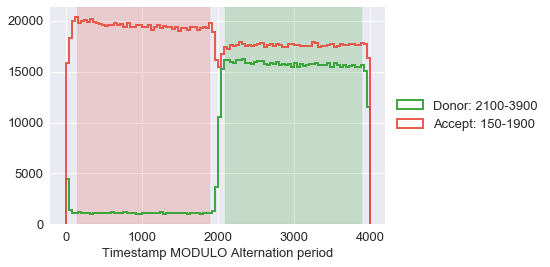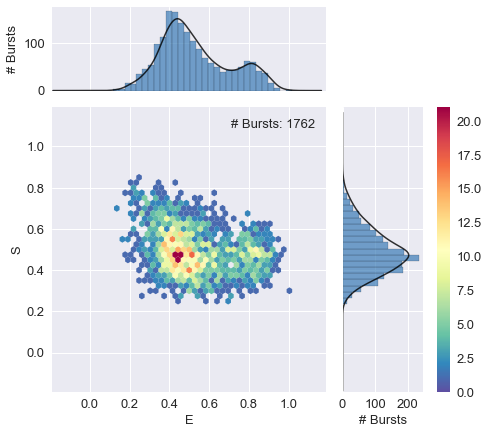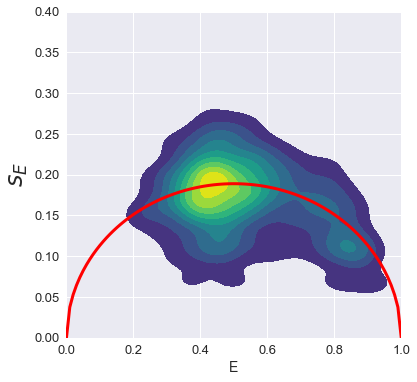# Example - Burst Variance Analysis¶

This notebook is part of smFRET burst analysis software FRETBursts.

This notebook shows how to implement Burst Variance Analysis (BVA) (Torella 2011) using FRETBursts.

For a complete tutorial on burst analysis see FRETBursts - us-ALEX smFRET burst analysis.

We start loading the FRETBursts software:

In :
from fretbursts import *
sns = init_notebook()

 - Optimized (cython) burst search loaded.
- Optimized (cython) photon counting loaded.
--------------------------------------------------------------
You are running FRETBursts (version 0.6.4).

If you use this software please cite the following paper:

FRETBursts: An Open Source Toolkit for Analysis of Freely-Diffusing Single-Molecule FRET
Ingargiola et al. (2016). http://dx.doi.org/10.1371/journal.pone.0160716

--------------------------------------------------------------


# Data file¶

In :
url = 'http://files.figshare.com/2182601/0023uLRpitc_NTP_20dT_0.5GndCl.hdf5'

URL:  http://files.figshare.com/2182601/0023uLRpitc_NTP_20dT_0.5GndCl.hdf5
File: 0023uLRpitc_NTP_20dT_0.5GndCl.hdf5

File already on disk: /Users/anto/src/FRETBursts/notebooks/data/0023uLRpitc_NTP_20dT_0.5GndCl.hdf5

In :
file_name = "0023uLRpitc_NTP_20dT_0.5GndCl.hdf5"

# Here the folder is the subfolder "data" of current notebook folder
folder_name = './data/'
full_fname = folder_name + file_name
full_fname

Out:
'./data/0023uLRpitc_NTP_20dT_0.5GndCl.hdf5'
In :
import os
if os.path.isfile(full_fname):
print ("Perfect, I found the file!")
else:
print ("Sorry, I can't find the file:\n", full_fname)

Perfect, I found the file!


# Load the selected file¶

In :
d = loader.photon_hdf5(full_fname)


# us-ALEX parameters¶

At this point, in d, we only have the timestamps (ph_times_t) and the detector numbers (det_t):

In :
d.add(det_donor_accept=(0, 1),
alex_period=4000,
D_ON=(2100, 3900),
A_ON=(150, 1900),
offset=700)
bpl.plot_alternation_hist (d)

Out:
data_0023uLRpitc_NTP_20dT_0.5GndCl G1.000# Burst Search and Selection¶

Here we perform a standard burst search using the donor-excitation photon stream (Ph_sel(Dex='DAem')) as required for BVA. Then, we apply a few selection filters to discard D-only and A-only bursts.

In :
d.calc_bg(bg.exp_fit, time_s=50.1, tail_min_us='auto', F_bg=1.7)

 - Calculating BG rates ... [DONE]

In :
d.burst_search(m=10, computefret=False, ph_sel=Ph_sel(Dex='DAem'))
d.calc_fret(count_ph=True, corrections=False)

 - Performing burst search (verbose=False) ... - Recomputing background limits for Dex ... [DONE]
- Recomputing background limits for all ... [DONE]
- Fixing  burst data to refer to ph_times_m ... [DONE]
[DONE]
- Calculating burst periods ...[DONE]

In :
ds = d.select_bursts(select_bursts.naa, th1=30, computefret=False)
ds1 = ds.select_bursts(select_bursts.size, th1=30, computefret=False)
ds_FRET = ds1.select_bursts(select_bursts.S, S1=0.25, S2=0.85, computefret=False)

In :
dx=ds_FRET
alex_jointplot(dx)

Out:
<seaborn.axisgrid.JointGrid at 0x118599cc0># Burst Variance Analysis¶

We define a function to compute $s_E$:

In :
def bva_sigma_E(n, bursts, DexAem_mask, out=None):
"""
Perform BVA analysis computing std.dev. of E for sub-bursts in each burst.

Split each burst in n-photons chunks (sub-bursts), compute E for each sub-burst,
then compute std.dev. of E across the sub-bursts.

For details on BVA see:

- Torella et al. (2011) Biophys. J. doi.org/10.1016/j.bpj.2011.01.066
- Ingargiola et al. (2016) bioRxiv, doi.org/10.1101/039198

Arguments:
n (int): number of photons in each sub-burst
bursts (Bursts object): burst-data object with indexes relative
to the Dex photon stream.
DexAem_mask (bool array): mask of A-emitted photons during D-excitation
periods. It is a boolean array indexing the array of Dex timestamps
(Ph_sel(Dex='DAem')).
out (None or list): append the result to the passed list. If None,
creates a new list. This is useful to accumulate data from
different spots in a single list.

Returns:
E_sub_std (1D array): contains for each burst, the standard deviation of
sub-bursts FRET efficiency. Same length of input argument bursts.
"""
E_sub_std = [] if out is None else out

for burst in bursts:
E_sub_bursts = []
startlist = range(burst.istart, burst.istop + 2 - n, n)
stoplist = [i + n for i in startlist]
for start, stop in zip(startlist, stoplist):
assert stop - start == n
E = A_D / n
E_sub_bursts.append(E)
E_sub_std.append(np.std(E_sub_bursts))

return E_sub_std


Next we prepare the data for BVA:

In :
ph_d = ds_FRET.get_ph_times(ph_sel=Ph_sel(Dex='DAem'))
bursts = ds_FRET.mburst
bursts_d = bursts.recompute_index_reduce(ph_d)

In :
Dex_mask = ds_FRET.get_ph_mask(ph_sel=Ph_sel(Dex='DAem'))


and call the bva_sigma_E function:

In :
n = 7
E_sub_std = bva_sigma_E(n, bursts_d, DexAem_mask_d)


Finally, we make a KDE plot of the 2D distribution E_sub_std versus the burst FRET efficiency:

In :
plt.figure(figsize=(6,6))
x = np.arange(0,1.01,0.01)
y = np.sqrt((x*(1-x))/n)
plt.plot(x,y, lw=3, color='red')
im = sns.kdeplot(ds_FRET.E, np.asfarray(E_sub_std), shade=True, cmap='viridis', shade_lowest=False)
plt.xlim(0,1)
plt.ylim(0,0.4)
plt.xlabel('E', fontsize=14)
plt.ylabel(r'$s_E$', fontsize=24);Executed: Tue Jul 11 21:51:51 2017

Duration: 7 seconds.

Autogenerated from: Example - Burst Variance Analysis.ipynb